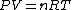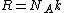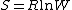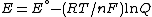# gas constant

Also found in: Dictionary, Thesaurus, Medical, Legal, Financial, Acronyms, Wikipedia.

## Gas constant

The universal constant R

(1)that appears in the ideal gas law, Eq. (1), where P is the pressure, V the volume, n the amount of substance, and T the thermodynamic (absolute) temperature. The gas constant is universal in that it applies to all gases, providing they are behaving ideally (in the limit of zero pressure). The gas constant is related to the more fundamental Boltzmann constant, k, by
(2)Eq. (2), where NA is the Avogadro constant (the number of entities per mole). The best modern value in SI units is R = 8.314 472 (15) J/K &cdot; mol, where the number in parentheses represents the uncertainty in the last two digits. See Boltzmann constant

According to the equipartition principle, at a temperature T, the average molar energy of each quadratic term in the expression for the energy is (1/2)RT; as a consequence, the translational contribution to the molar heat capacity of a gas at constant volume is (3/2)R; the rotational contribution of a linear molecule is R. See Kinetic theory of matter

Largely because R is related to the Boltzmann constant, it appears in a wide variety of contexts, including properties unrelated to gases. Thus, it occurs in Boltzmann's formula for the molar entropy of any substance, Eq. (3),

(3)where W is the number of arrangements of the system that are consistent with the same energy; and in the Nernst equation for the potential of an electrochemical cell, Eq. (4),
(4)where E° is a standard potential, F is the Faraday constant, and Q is a function of the composition of the cell. The gas constant also appears in the Boltzmann distribution for the population of energy levels when the energy of a level is expressed as a molar quantity. See Boltzmann statistics, Entropy

McGraw-Hill Concise Encyclopedia of Physics. © 2002 by The McGraw-Hill Companies, Inc.

## gas constant

[′gas ‚kän·stənt]
(thermodynamics)
The constant of proportionality appearing in the equation of state of an ideal gas, equal to the pressure of the gas times its molar volume divided by its temperature. Also known as gas-law constant; universal gas constant.
McGraw-Hill Dictionary of Scientific & Technical Terms, 6E, Copyright © 2003 by The McGraw-Hill Companies, Inc.
References in periodicals archive ?
where [[eta].sub.act] is activation voltage, [[alpha].sub.c] is load transport coefficient of the cathode side, F is the Faraday constant, R is the universal gas constant, T is fuel cell operating temperature,
Q and R are the activation energy and gas constant of the solder.
where constant [B.sub.T] = RT/[b.sub.T], which is related to the adsorption heat, R is the gas constant (8.314 J/mol K), T (K) is absolute temperature in Kelvin, [b.sub.T] (J/mol) is the Temkin isotherm constant, which is the variation of adsorption energy and [K.sub.T] is the equilibrium binding constant corresponding to the maximum binding energy.
where [E.sub.a] is the activation energy of diffusion, R is the ideal gas constant, T is the given temperature, and [T.sub.ref] is is a reference temperature.
(2000) in Their Fundamental Equation for Dry Air Quantity Symbol Value Universal molar [[bar.R].sup.Lem] 8.314510 gas constant kJ/(kmol*K) Specific gas [R.sup.Lem] 0.287117 constant kJ/(kg*K) Molar mass [M.sup.Lem] 28.9586 kg/kmol Maxcondentherm [[bar.[rho]].sub.j] 10.4477 molar density mol/[dm.sup.3] Maxcondentherm [T.sub.j] 132.6312 K temperature The dimensionless form of the fundamental equation reads
NOMENCLATURE [C.sub.1] constant defined by Equations (8) or (9) ([Pa.sup.-1]) [C.sub.2] constant defined by Equations (8) or (10) ([Pa.sup.-1]) D diffusion coefficient of gas in tube ([m.sup.2]/s) L length of the collector tube (m) M molecular weight (kg/mol) N number of moles (mol) p pressure (Pa) Q gas flow rate (mol/s) r internal radius of tube (m) R universal gas constant (J/mol K) t time (s) T absolute temperature (K) V volume of the system ([m.sup.3]) x position within the collector tube (m) Greek Symbols [eta] dynamic viscosity of the gas (kg/m s) [[lambda].sub.n] eigenvalue in Equation (17) [tau] dimensionless time defined by Equation (18) (-)
By comparison with the theory of elasticity, it is proposed that [C.sub.1] = 0.5NRT, where N = crosslink density, R = gas constant and T = absolute temperature.
(Where R is the gas constant (R=1.987x10-3 kcal/mol/K) and T is the absolute temperature in Kelvin as T=298 K)
For gases the accepted difference between molar isobaric heat capacity and molar isometric heat capacity is the ideal gas constant (R).
Where k 2 is the pseudo second order rate constant, A is pre-exponential factor of the Arrhenius equation, E a is the activation energy of the reaction, R is gas constant (8.314 Jmol -1 K -1 ), T is absolute temperature in K, C i is initial concentration before adsorption and C f is concentration left in solution after adsorption.
where [C.sub.A] and [C.sub.B] are the concentrations (mol/[m.sup.3]) of species in the reactant side, A is the pre- exponential factor ([s.sup.-1]), [E.sub.a] is activation energy (J [mol.sup.-1]) and R is universal gas constant (J [mol.sup.-1] [K.sup.-1]).
In this equation, A is the pre-exponential factor [E.sub.A] is the activation energy, R is the molar gas constant and T is the ageing temperature.

Site: Follow: Share:
Open / Close